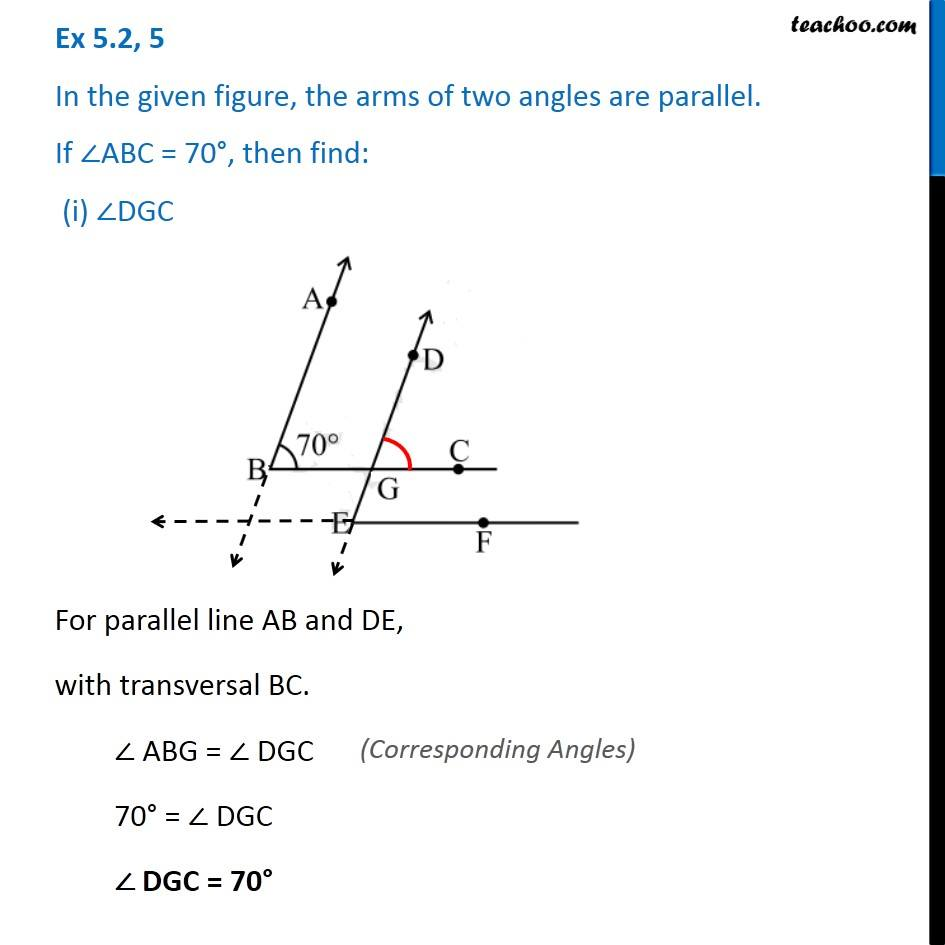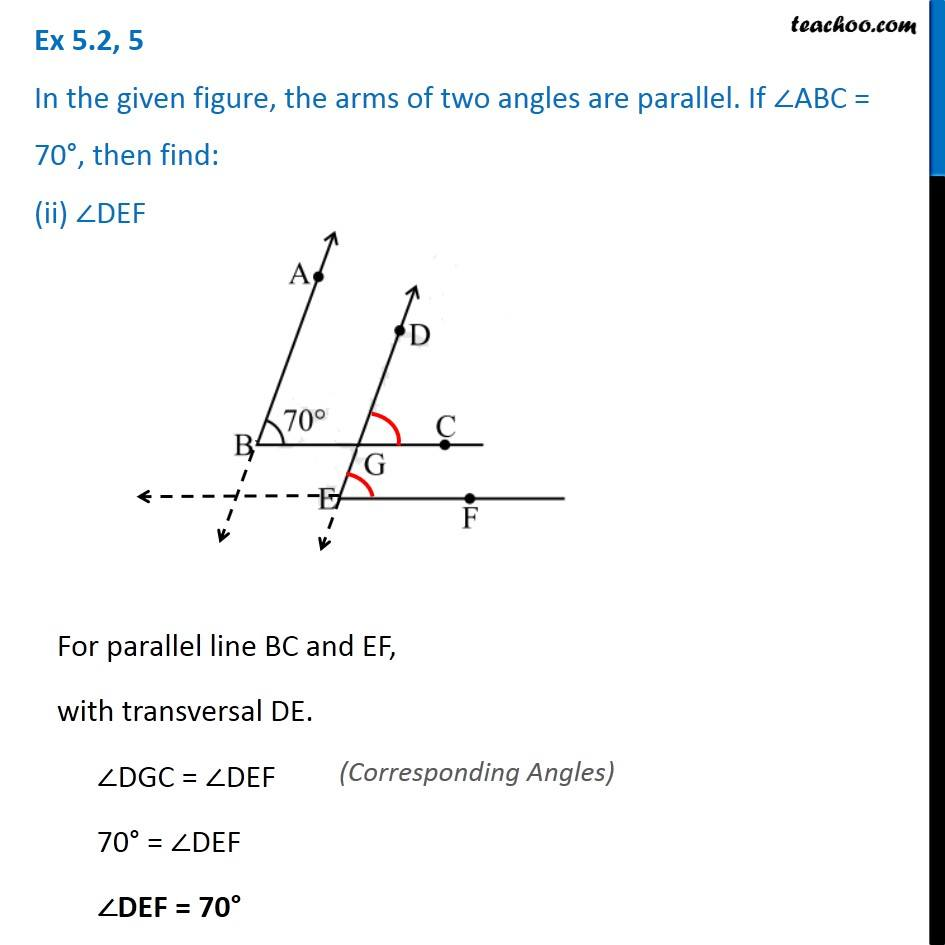Ex 5.2

Chapter 5 Class 7 Lines and Angles
Serial order wiseLearn in your speed, with individual attention - Teachoo Maths 1-on-1 Class

### Transcript

Ex 5.2, 5 In the given figure, the arms of two angles are parallel. If ∠ABC = 70°, then find: (i) ∠DGC For parallel line AB and DE, with transversal BC. ∠ ABG = ∠ DGC 70° = ∠ DGC ∠ DGC = 70° Ex 5.2, 5 In the given figure, the arms of two angles are parallel. If ∠ABC = 70°, then find: (ii) ∠DEFFor parallel line BC and EF, with transversal DE. ∠DGC = ∠DEF 70° = ∠DEF ∠DEF = 70°